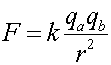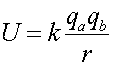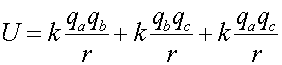Electric Potential Energy

Just like in the gravitational case, the potential falls proportional to 1/r. The form of the potential energy U looks the same as the that for the force F except for the different power of r.You include the signs of the charges when you use this formula (unlike the case of calculating the magnitude of a force), so the potential energy is positive if the two charges have the same sign and negative otherwise.

The potential energy of a set of charges, qa,qb,qc,... is the sum of the potential energies of the pairs. For instance, if there are 3 charges, the net potential energy is:Potential energy is NOT a vector quantity, so the contributions from various charges add up algebraically in this simple way.

Examples       Coulomb's law index